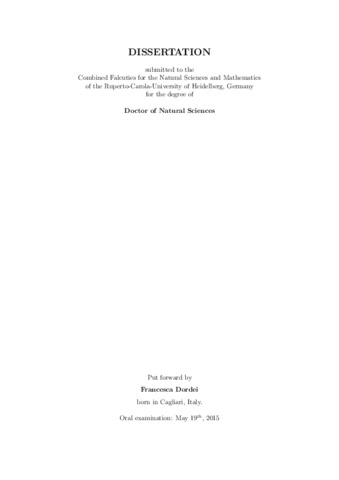Dordei, FrancescaPreview
PDF, English

Citation of documents: Please do not cite the URL that is displayed in your browser location input, instead use the DOI, URN or the persistent URL below, as we can guarantee their long-time accessibility.

## Abstract

This thesis presents several lifetime measurements of b-flavoured hadrons at the LHCb experiment. They represent an important test of the theoretical approach to b-hadron observables known as HeavyQuark Expansion (HQE). This analysis uses data corresponding to an integrated luminosity of 1 fb-1 collected in proton-proton collisions at a centre-of-mass energy of 7 TeV. For the decays B+ -> J/psi K+, B0 -> J/psi K*0, B0 -> J/psi K0S, Lambdab -> J/psi Lambda and B0s -> J/psi phi the lifetimes are measured to be

tau(B+ -> J/psi K+) = 1.637 +- 0.004 +- 0.003 ps, tau(B0 -> J/psi K*0) = 1.524 +- 0.006 +- 0.004 ps, tau(B0 -> J/psi K0S) = 1.499 +- 0.013 +- 0.005 ps, tau(Lambdab -> J/psi Lambda) = 1.415 +- 0.027 +- 0.006 ps, tau(B0s -> J/psi phi) = 1.480 +- 0.011 +- 0.005 ps,

where the first uncertainty is statistical and the second is systematic. These are the most precise lifetime measurements in these decay modes to date. Ratios of these lifetimes also are reported in this thesis, as they are well-defined quantities where many theoretical or experimental uncertainties cancel. The ratio of the decay width difference, Delta Gamma_d, to the average width, Gamma_d, in the B0 system is found to be Delta Gamma_d/ Gamma_d = - 0.044 +- 0.025 +- 0.011. Using data corresponding to an integrated luminosity of 3 fb-1 collected at centre-of-mass energies of 7 TeV and 8 TeV, the measurements of the decay width difference, Delta Gamma_s, and the average width, Gamma_s, in the B0s system are performed. Using the decay B0s -> J/psi phi, they are measured to be

Delta Gamma_s = 0.0805 +- 0.0091 +- 0.0033 ps-1, Gamma_s = 0.6603 +- 0.0027 +- 0.0015 ps-1.

These are the most precise determinations of these observables ot date. All measurements are consistent with HQE predictions.

Item Type: Dissertation Hansmann-Menzemer, Prof. Dr. Stephanie Heidelberg 19 May 2015 29 May 2015 10:48 2015 The Faculty of Physics and Astronomy > Dekanat der Fakultät für Physik und AstronomieThe Faculty of Physics and Astronomy > Institute of Physics 530 Physics